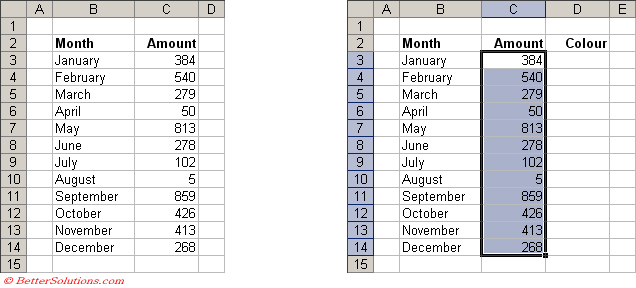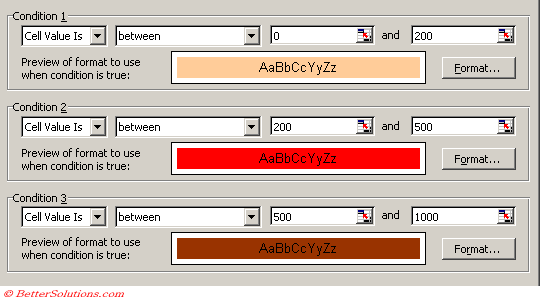# Sorting

You cannot currently sort a cell range based on the conditional formatting, however an alternative is to insert the same condition in the corresponding row or column, so it returns True or False. You can then sort by this row or column.
SS

You can use conditional formatting as a way to check that the data is sorted correctly.
Have 2 conditions A2<A1 and A2 > A1

### Using Manual Formatting

If you want to sort using the background colour of the cells you can refer to the Interior property of the Range object

`Range("C3").Interior.ColorIndex `

If you want to sort using the colour of the text you can use the Font property of the Range object.

`Range("C3").Font.ColorIndex `

For more details please refer to the Sorting by Colour page.

Lets assume that you have a simple table of data and that the formatting has been applied using Conditional Formatting.
This extra column will contain a user defined function which can be used to indicate the order in which to sort the rows.### Enter Conditions

Select the cells you want to apply the conditional formatting to, in this case "C3:C14".
Press (Format > Conditional Formatting) to display the Conditional Formatting dialog box.
Enter the three conditions, we have used constants to keep it simple.### Conditional Formatting

If a cell has been formatted with Conditional Formatting then using the ColorIndex of the cell will not work.
The following two properties are undefined when you have applied conditional formatting.

`Range("C3").Interior.ColorIndex Range("C3").Font.ColorIndex `

The only way to determine the type of formatting applied is to analyse the Conditional Formatting directly.

`Dim objFormatConditions As FormatConditions objFormatConditions(1).Interior.ColorIndex objFormatConditions(1).Font.ColorIndex `

### User Defined Function

This user defined function will return the colour index of the cell reference which is passed as the first argument.
The number returned will always be between 1 and 52 since this corresponds to the location on the colour palette.
Once the user defined function has been entered into a code module in the corresponding VBA Project you can use it in your extra column.
If the cell does not contain any conditional formatting then 0 is returned.

`Option Explicit Public Function ColourSorting(ByVal rgeCell As Range, _                               ByVal bBackGround As Boolean, _                               ByVal bText As Boolean) As Integer Dim iconditionno As Integer    If rgeCell.FormatConditions.Count = 0 Then Exit Function    iconditionno = ConditionNo(rgeCell)    If bBackGround = True Then       ColourSorting = rgeCell.FormatConditions(iconditionno).Interior.ColorIndex    End If    If bText = True Then       ColourSorting = rgeCell.FormatConditions(iconditionno).Font.ColorIndex    End If End Function `

`Private Function ConditionNo(ByVal rgeCell As Range) As Integer Dim iconditionscount As Integer Dim objFormatCondition As FormatCondition     For iconditionscount = 1 To rgeCell.FormatConditions.Count         Set objFormatCondition = rgeCell.FormatConditions(iconditionscount)         Select Case objFormatCondition.Type            Case xlCellValue                Select Case objFormatCondition.Operator                    Case xlBetween:      If Compare(rgeCell.Value, ">=", objFormatCondition.Formula1) = True And _                                            Compare(rgeCell.Value, "<=", objFormatCondition.Formula2) = True Then _                                            ConditionNo = iconditionscount                                          Case xlNotBetween:   If Compare(rgeCell.Value, "<=", objFormatCondition.Formula1) = True And _                                            Compare(rgeCell.Value, ">=", objFormatCondition.Formula2) = True Then _                                            ConditionNo = iconditionscount                       Case xlGreater:      If Compare(rgeCell.Value, ">", objFormatCondition.Formula1) = True Then _                                            ConditionNo = iconditionscount                                           Case xlEqual:        If Compare(rgeCell.Value, "=", objFormatCondition.Formula1) = True Then _                                            ConditionNo = iconditionscount                         Case xlGreaterEqual: If Compare(rgeCell.Value, ">=", objFormatCondition.Formula1) = True Then _                                            ConditionNo = iconditionscount                         Case xlLess:         If Compare(rgeCell.Value, "<", objFormatCondition.Formula1) = True Then _                                            ConditionNo = iconditionscount                                           Case xlLessEqual:    If Compare(rgeCell.Value, "<=", objFormatCondition.Formula1) = True Then _                                            ConditionNo = iconditionscount                                                                       Case xlNotEqual:     If Compare(rgeCell.Value, "<>", objFormatCondition.Formula1) = True Then _                                            ConditionNo = iconditionscount                                                             If ConditionNo > 0 Then Exit Function               End Select           Case xlExpression             If Application.Evaluate(objFormatCondition.Formula1) Then                ConditionNo = iconditionscount                Exit Function             End If        End Select     Next iconditionscount End Function `

`Private Function Compare(ByVal vValue1 As Variant, _                          ByVal sOperator As String, _                          ByVal vValue2 As Variant) As Boolean                           If Left(CStr(vValue1), 1) = "=" Then vValue1 = Range(Mid(CStr(vValue1), 2)).Value    If Left(CStr(vValue2), 1) = "=" Then vValue2 = Range(Mid(CStr(vValue2), 2)).Value                           If IsNumeric(vValue1) = True Then vValue1 = CDbl(vValue1)    If IsNumeric(vValue2) = True Then vValue2 = CDbl(vValue2)       Select Case sOperator       Case "=": Compare = (vValue1 = vValue2)       Case "<": Compare = (vValue1 < vValue2)       Case "<=": Compare = (vValue1 <= vValue2)       Case ">": Compare = (vValue1 > vValue2)       Case ">=": Compare = (vValue1 >= vValue2)       Case "<>": Compare = (vValue1 <> vValue2)    End Select End Function `